# Standard Algorithm

Page 2 of 5

Here's another one: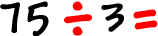Set it up:Remember, the DMS loop!  Division, then multiplication, then subtraction...  and we do this until we run out of numbers!

Division:

We want to see how many times 3 will go into 7...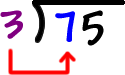Hmm...  It doesn't go in cleanly does it?  From our times tables, we know that 3 goes into 6 and 3 goes into 9.  But, 3 doesn't go into 7.  That's OK.

So, what does 3 go into that's a little less than 7?   SIX  Three goes into 6 two times.

 So, 3 goes into 7 two times... with a little left over.  Put that 2 right above the 7: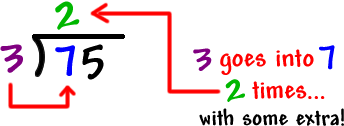OK, that was a little freaky, but it won't get any worse.  You'll get it!  Let's keep going!

 Multiplication: Multiply the 2 and the 3 and put the answer right under the 7: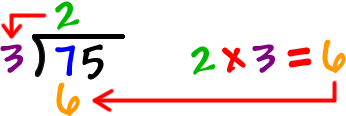Subtraction: Do the subtraction...That's 7 - 6 = 1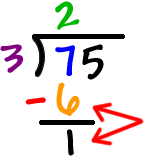Hey, we just finished the first chunk of steps!  And it wasn't that bad!

DMS!
Division, multiplication, subtraction.

OK, now we're going to do the exact same thing, but with a different number...

 The first thing is to set it up:  Drag the 5 down. That makes it a 15...Now, let's go back into our DMS loop using the 15!

 Division: We want to see how many times 3 will go into 15...  3 goes into 15 five times...  So, we put that 5 right above the 5: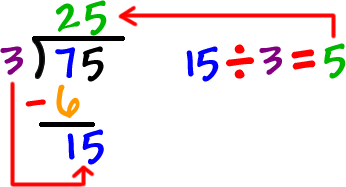Multiplication: Multiply the 5 and the 3 and put the answer right under the 15: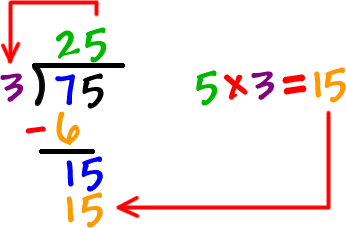Subtraction: That's 15 - 15 = 0 We've use all the numbers...  the 7 and the 5... and we got a 0 at the end... DONE!  Woo hoo! The answer is 25.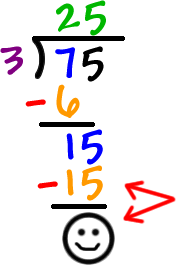Check it!!  25 x 3 should = 75!  Yep!

Go to the next page for another one!